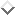Next: Newton's Laws of Motion Up: Newton's Laws of Motion Previous: Introduction

Newtonian Dynamics

Newtonian dynamics is a mathematical model whose purpose is to predict the motions of the various objects that we encounter in the world around us. The general principles of this model were first enunciated by Sir Isaac Newton in a work entitled Philosophiae Naturalis Principia Mathematica (Mathematical Principles of Natural Philosophy). This work, which was first published in 1687, is nowadays more commonly referred to as the Principa.Up until the beginning of the 20th century, Newton's theory of motion was thought to constitute a complete description of all types of motion occurring in the Universe. We now know that this is not the case. The modern view is that Newton's theory is only an approximation which is valid under certain circumstances. The theory breaks down when the velocities of the objects under investigation approach the speed of light in vacuum, and must be modified in accordance with Einstein's special theory of relativity. The theory also fails in regions of space which are sufficiently curved that the propositions of Euclidean geometry do not hold to a good approximation, and must be augmented by Einstein's general theory of relativity. Finally, the theory breaks down on atomic and subatomic length-scales, and must be replaced by quantum mechanics. In this book, we shall neglect relativistic and quantum effects all together. It follows that we must restrict our investigations to the motions of large (compared to an atom) slow (compared to the speed of light) objects moving in Euclidean space. Fortunately, virtually all of the motions which we commonly observe in the world around us fall into this category.

Newton very deliberately modeled his approach in the Principia on that taken in Euclid's Elements. Indeed, Newton's theory of motion has much in common with a conventional axiomatic system such as Euclidean geometry. Like all such systems, Newtonian dynamics starts from a set of terms that are undefined within the system. In this case, the fundamental terms are mass, position, time, and force. It is taken for granted that we understand what these terms mean, and, furthermore, that they correspond to measurable quantities which can be ascribed to, or associated with, objects in the world around us. In particular, it is assumed that the ideas of position in space, distance in space, and position as a function of time in space, are correctly described by the Euclidean vector algebra and vector calculus discussed in the previous chapter. The next component of an axiomatic system is a set of axioms. These are a set of unproven propositions, involving the undefined terms, from which all other propositions in the system can be derived via logic and mathematical analysis. In the present case, the axioms are called Newton's laws of motion, and can only be justified via experimental observation. Note, incidentally, that Newton's laws, in their primitive form, are only applicable to point objects. However, these laws can be applied to extended objects by treating them as collections of point objects.

One difference between an axiomatic system and a physical theory is that, in the latter case, even if a given prediction has been shown to follow necessarily from the axioms of the theory, it is still incumbent upon us to test the prediction against experimental observations. Lack of agreement might indicate faulty experimental data, faulty application of the theory (for instance, in the case of Newtonian dynamics, there might be forces at work which we have not identified), or, as a last resort, incorrectness of the theory. Fortunately, Newtonian dynamics has been found to give predictions which are in excellent agreement with experimental observations in all situations in which it is expected to be valid.

In the following, it is assumed that we know how to set up a rigid Cartesian frame of reference, and how to measure the positions of point objects as functions of time within that frame. It is also taken for granted that we have some basic familiarity with the laws of mechanics, and standard mathematics up to, and including, calculus, as well as the vector analysis outlined in Appendix A.Next: Newton's Laws of Motion Up: Newton's Laws of Motion Previous: Introduction
Richard Fitzpatrick 2011-03-31